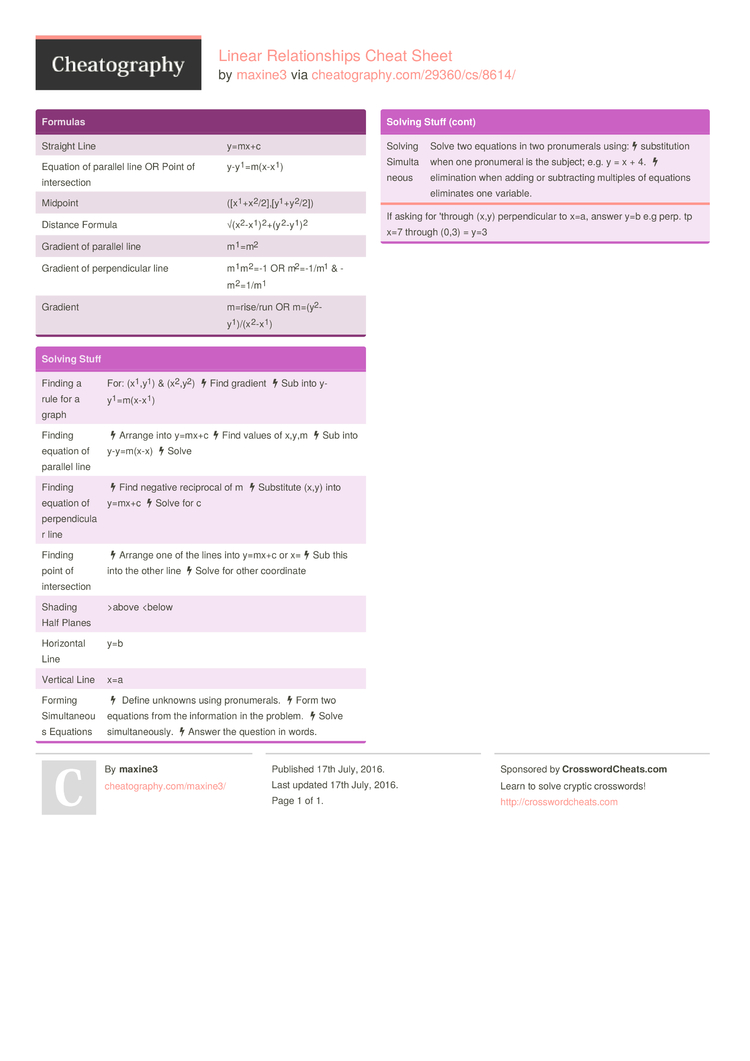Cheatography

# Linear Relationships Cheat Sheet by maxine3

MATHSSSSSSSSSSSSSSSSSSSSS

### Formulas

 Straight Line y=mx+c Equation of parallel line OR Point of inters­ection y-y1­=m(­x-x1) Midpoint ([x1­+x­2/­2],­[y­1+­y2­/2]) Distance Formula √(x2­-x­1)­2­+(y­2­-y­1)2 Gradient of parallel line m1=m2 Gradient of perpen­dicular line m1m­2=-1 OR m2=-­1/m1 & -m2=­1/m1 Gradient m=rise/run OR m=(y2­-y­1­)/(­x2­-x1)

### Solving Stuff

 Finding a rule for a graph For: (x1,­y1) & (x2,­y2) Find gradient Sub into y-y1­=m(­x-x1) Finding equation of parallel line Arrange into y=mx+c Find values of x,y,m Sub into y-y=m(x-x) Solve Finding equation of perpen­dicular line Find negative reciprocal of m Substitute (x,y) into y=mx+c Solve for c Finding point of inters­ection Arrange one of the lines into y=mx+c or x= Sub this into the other line Solve for other coordinate Shading Half Planes >above
If asking for 'through (x,y) perpen­dicular to x=a, answer y=b e.g perp. tp x=7 through (0,3) = y=31 Page
//media.cheatography.com/storage/thumb/maxine3_linear-relationships.750.jpg

PDF (recommended)